# SSC CHSL Simple and Compound Interest Questions

SSC CHSL Simple and Compound Interest Questions with Solutions for Competitive Exams. Question answer practice set from Previous year question paper for the self study of upcoming exams.

EXAM : SSC CHSL (AUGUST 2021 ALL SHIFT)
Medium : ENGLISH

## Simple and Compound Interest Questions

Q.1: Varun and Madhur invested ₹25,000 each in different schemes. Varun earned simple interest at 11% per annum, whereas Madhur earned compound interest at 10% per annum compounded annually. Who received more interest after 2 years and how much?
(A) Varun, ₹250
(C) Varun, ₹500

Ans : (A) Varun, ₹250
Varun’s simple interest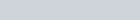$=\frac{25000\times11\times2}{100} = 5500$
Madhur’s compound interest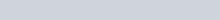$C.I.=A - P(1+\frac{R}{100})^n=5250$
Profit of varun =5500 – 5250 = 250₹

Q.2: If the difference between the compound interest compounded annually and the simple interest on a certain sum of money for three years at 10% per annum ₹279, then the sum ( in ₹ ) is:
(A) 10,000
(B) 9,000
(C) 8,000
(D) 7,500

Ans : (B) 9,000
Of 3 years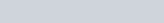$C.I. - S.I. = \frac{Pr^2 (300+r)}{1000000}$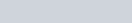$279 =\frac{P\times100 ( 300+10)}{1000000}$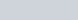$P=\frac{2790000}{310}$
P = 9000₹

Q.3: What will be the compound interest ( in ₹ ) on a sum of ₹7,200 for 18 months at a rate of 20% per annum, if the interest is compounded half-yearly (nearest to an integer) ?
(A) 2,833
(B) 2,383
(C) 2,338
(D) 3,238

Ans : (B) 2,383
P = 7200
R% = 20% (Annual)
R% = 10% (Half yearly)
T = 18 months = 3 half yearly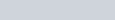$A=P( 1+\frac{R}{100})^n$
total C.I. = 2383.2 = 2383₹

Q.4: A sum fo money lent at simple interest amounts to ₹9,920 after 2 years and to ₹12,800 after 5 years. Find the rate of interest per annum.
(A) 18%
(B) 9.68%
(C) 12%
(D) 6.57%

Ans : (C) 12%
2 year compound = 9920
5 year compound = 12800
3 year interest = 2880₹
1 year simple interest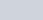$=\frac{2880}{3}$
1 year S.I. = 960₹
2 year S.I. = 1920
Principal = 9920 – 1920 = 8000
Rate (R)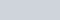$=\frac { SI\times100}{ P\times T}$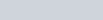$=\frac{960\times100}{8000\times1}=12$%

Q.5: The 12% interest earned on a sum of ₹2,60,000 is to be used for awarding 24 scholarship of equal value every year in a school. What is the value ( in ₹) of each scholarship?
(A) 1,250
(B) 1,200
(C) 1,150
(D) 1,300

Ans : (D) 1,300
Simple interest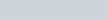$(S. I.)=\frac{ P \times R \times T }{100}$
Value of each scholarship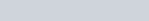$=\frac{260,000\times12\times1}{100\times24} = 1300$

Q.6: If the simple interest on a certain sum of money borrowed for 4 years at 9.5% per annum exceeds the simple interest on the same sum for 3 years 12.5% per annum by ₹225, then the sum borrowed is:
(A) ₹35,000
(B) ₹45,000
(C) ₹42,000
(D) ₹48,000

Ans : (B) ₹45,000
R% x T = S.I.
9.5% x 4 = 38%
12.5% x 3 = 37.5%
Difference in interest = 38% – 37.5%
0.5% = 225
1% =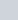$\frac{225}{0.5}$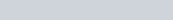$100=\frac{225}{0.5}\times100 = 45000$

Q.7: The compound interest on a certain sum of money for 3 years, compounded annually, at a rate of interest of 10% per annum is ₹1,324. The sum is:
(A) ₹4,000
(B) ₹5,500
(C) ₹5,000
(D) ₹4,500

Ans : (A) ₹4,000
R% = 10% =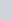$\frac{1}{10}$
10 : 11
10 : 11
10 : 11
1000 : 1331
(C.I.) = 1331 – 1000 = 331
A.T.Q. 331 = 1324
1 = 4
Principal (Amount) = 4 x 1000 = 4000

Thanks for attempt SSC CHSL Simple and Compound Interest Questions for competitive exams.

Simple and Compound Interest Questions of SSC CHSL in Hindi – SSC STUDY

## Maths Practice Set for Competitive Exams

Simple and Compound Interest Questions with Answers

Time and Work Questions with Answers